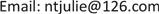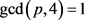﻿ 完全二部多重图的K2,4-因子分解 K2,4-Factorization of Complete Bipartite Multigraphs

Pure Mathematics
Vol. 09  No. 02 ( 2019 ), Article ID: 29331 , 6 pages
10.12677/PM.2019.92023

K2,4-Factorization of Complete Bipartite Multigraphs

Li Zhu

Nantong Vocational University, Nantong JiangsuReceived: Feb. 26th, 2019; accepted: Mar. 13th, 2019; published: Mar. 20th, 2019ABSTRACT

Let $\lambda {K}_{m}{}_{,n}$ be a complete bipartite multigraph with two partite sets having m and n vertices, respectively. A ${K}_{p}{}_{,q}$ -factorization $\lambda {K}_{m}{}_{,n}$ is a set of edge-disjoint ${K}_{p}{}_{,q}$ -factors of $\lambda {K}_{m}{}_{,n}$ . When p = 1, q = 2 and p = 2, q = 3, the ${K}_{p}{}_{,q}$ -factorization of $\lambda {K}_{m}{}_{,n}$ has been completely solved. When p = 1, q = 3 and p = 1, q = 4, the ${K}_{p}{}_{,q}$ -factorization of ${K}_{m}{}_{,n}$ has been totally solved. In this article, the K2,4-factorization of $\lambda {K}_{m}{}_{,n}$ is researched. We will give a necessary and sufficient condition for K2,4-factorization of $\lambda {K}_{m}{}_{,n}$ , that is: 1) $m\equiv n\equiv 0$ (mod 2), 2) m £ 2n, 3) n £ 2m, 4) $m+n\equiv 0$ (mod 6), 5) $3\lambda mn/\left[4\left(m+n\right)\right]$ .

Keywords:Complete Bipartite Multigraphs, Factor, Factorization1. 引言

Km,n表示完全二部图，其两个部分点集Ｘ和Ｙ分别具有m和n个点。lKm,n表示完全二部多重图，它是l个两两不交的同构于Km,n的图的并。如果lKm,n的一个子图F包含了lKm,n的所有点，则称Ｆ为lKm,n的一个支撑子图。若lKm,n的支撑子图F的每个分支均同构于图Kp,q，则称F为lKm,n的一个Kp,q-因子。如果lKm,n的边集可以划分为lKm,n的Kp,q-因子，则称lKm,n存在Kp,q-因子分解。在综述文章  中，Ushio称lKm,n的Kp,q-因子分解为可分解的(m, n, k, l)二部Kp,q-设计。如果lKm,n存在Kp,q-因子分解，则称lKm,n是可Kp,q-因子分解的。本文用到的图论方面的名词术语，均参照图论著作  。

lKm,n的Kp,q-因子分解有许多应用，特别是Yamamoto和Ushio等  用其建立了计算机数据存储的HUBMFS2方案。当p = 1和q = 2时，Ushio  、Wang和Du  完全解决了lKm,n的K1,2-因子分解的存在性问题。Martin在论文   中分别解决了当p = 1、q = 3与p = 1、q = 4时Km,n的Kp,q-因子分解的存在性。当p = 2和q = 3时，Wang和Du  完全解决了l = 1时Km,n的K2,3-因子分解的存在性。我们在论文  中完全解决了l > 1时lKm,n的K2,3-因子分解的存在性。本文研究当p = 2和q = 4时，完全二部多重图lKm,n的K2,4-因子分解的存在性。即我们将证明lKm,n存在K2,4-因子分解的充分必要条件。

2. 定理1.1的证明

$d=4\left(2p+q\right)z/\left(\lambda pq\right)$

$m=4\left(p+q\right)\left(\text{2}p+q\right)z/\left(\lambda pq\right)$

$n=2\left(\text{4}p+q\right)\left(\text{2}p+q\right)z/\left(\lambda pq\right)$

$r=\left(p+q\right)\left(4p+q\right)z/\left(\lambda pq\right)$

$a=\text{2}p\left(\text{2}p+q\right)z/\left(\lambda pq\right)$

$b=q\left(\text{2}p+q\right)z/\left(\lambda pq\right)$

$d=\text{4}\left(\text{2}p+q\right)s/\gamma ,m=\text{4}\left(p+q\right)\left(\text{2}p+q\right)s/\gamma ,n=\text{2}\left(\text{4}p+q\right)\left(\text{2}p+q\right)s/\gamma$

$r=\left(p+q\right)\left(\text{4}p+q\right)s\lambda /\gamma ,a=\text{2}p\left(\text{2}p+q\right)s/\gamma ,b=q\left(\text{2}p+q\right)s/\gamma$

2) 如果$\mathrm{gcd}\left(q,16\right)=1$，设 $q=\text{2}{q}_{\text{1}}$，设 $\mathrm{gcd}\left(2\left(p+{q}_{1}\right),\lambda \right)=\gamma$，则

$d=\text{4}\left(p+{q}_{\text{1}}\right)s/\gamma ,m=\text{4}\left(p+\text{2}{q}_{\text{1}}\right)\left(p+{q}_{\text{1}}\right)s/\gamma ,n=\text{4}\left(\text{2}p+{q}_{\text{1}}\right)\left(p+{q}_{\text{1}}\right)s/\gamma$

$r=\left(p+\text{2}{q}_{\text{1}}\right)\left(\text{2}p+{q}_{\text{1}}\right)s\lambda /\gamma ,a=\text{2}p\left(p+{q}_{\text{1}}\right)s/\gamma ,b=\text{2}{q}_{\text{1}}\left(p+{q}_{\text{1}}\right)s/\gamma$

3) 如果 $\mathrm{gcd}\left(p,4\right)=1$$\mathrm{gcd}\left(q,16\right)=\text{4}$，设 $q=4{q}_{2}$，设 $\mathrm{gcd}\left(p+2{q}_{2},l\right)=\gamma$，则

$d=\text{2}\left(p+\text{2}{q}_{\text{2}}\right)s/\gamma ,m=\text{2}\left(p+\text{4}{q}_{\text{2}}\right)\left(p+\text{2}{q}_{\text{2}}\right)s/\gamma ,n=\text{4}\left(p+{q}_{\text{2}}\right)\left(p+\text{2}{q}_{\text{2}}\right)s/\gamma$

$r=\left(p+\text{4}{q}_{\text{2}}\right)\left(p+{q}_{\text{2}}\right)s\lambda /\gamma ,a=p\left(p+\text{2}{q}_{\text{2}}\right)s/\gamma ,b=\text{2}{q}_{\text{2}}\left(p+\text{2}{q}_{\text{2}}\right)s/\gamma$

4) 如果 $\mathrm{gcd}\left(p,4\right)=1$$\mathrm{gcd}\left(q,16\right)=8$，设 $q=8{q}_{3}$，设 $\mathrm{gcd}\left(p+4{q}_{3},\lambda \right)=\gamma$，则

$d=\text{2}\left(p+\text{4}{q}_{\text{3}}\right)s/\gamma ,m=\text{2}\left(p+\text{8}{q}_{\text{3}}\right)\left(p+\text{4}{q}_{\text{3}}\right)s/\gamma ,n=\text{4}\left(p+\text{2}{q}_{\text{3}}\right)\left(p+\text{4}{q}_{\text{3}}\right)s/\gamma$

$r=\left(p+\text{8}{q}_{\text{3}}\right)\left(p+\text{2}{q}_{\text{3}}\right)s\lambda /\gamma ,a=p\left(p+\text{4}{q}_{\text{3}}\right)s/\gamma ,b=\text{4}{q}_{\text{3}}\left(p+\text{4}{q}_{\text{3}}\right)s/\gamma$

5) 如果 $\mathrm{gcd}\left(p,4\right)=1$$\mathrm{gcd}\left(q,16\right)=16$，设 $q=16{q}_{4}$，设 $\mathrm{gcd}\left(p+8{q}_{4},\lambda \right)=\gamma$，则

$d=\text{2}\left(p+\text{4}{q}_{\text{4}}\right)s/\gamma ,m=\text{2}\left(p+\text{16}{q}_{\text{4}}\right)\left(p+\text{8}{q}_{\text{4}}\right)s/\gamma ,n=\text{4}\left(p+\text{4}{q}_{\text{4}}\right)\left(p+\text{8}{q}_{\text{4}}\right)s/\gamma$

$r=\left(p+\text{16}{q}_{\text{4}}\right)\left(p+\text{4}{q}_{\text{4}}\right)s\lambda /\gamma ,a=p\left(p+\text{8}{q}_{\text{4}}\right)s/\gamma ,b=\text{8}{q}_{\text{4}}\left(p+\text{8}{q}_{\text{4}}\right)s/\gamma$

6) 如果 $\text{gcd}\left(p,\text{4}\right)=\text{2}$$\text{gcd}\left(q,\text{16}\right)=\text{1}$，设 $p=2{p}_{1}$，设 $\mathrm{gcd}\left(4{p}_{1}+q,\lambda \right)=\gamma$，则

$d=4\left(4{p}_{1}+q\right)s/\gamma ,m=4\left(2{p}_{1}+q\right)\left(4{p}_{1}+q\right)s/\gamma ,n=2\left(8{p}_{1}+q\right)\left(4{p}_{1}+q\right)s/\gamma$

$r=\left(2{p}_{1}+q\right)\left(8{p}_{1}+q\right)s\lambda /\gamma ,a=4{p}_{1}\left(4{p}_{1}+q\right)s/\gamma ,b=q\left(4{p}_{1}+q\right)s/\gamma$

7) 如果 $\mathrm{gcd}\left(p,4\right)=4$$\mathrm{gcd}\left(q,16\right)=1$，设 $p=4{p}_{2}$，设 $\mathrm{gcd}\left(8{p}_{2}+q,\lambda \right)=\gamma$，则

$d=4\left(8{p}_{2}+q\right)s/\gamma ,m=4\left(4{p}_{2}+q\right)\left(8{p}_{2}+q\right)s/\gamma ,n=2\left(16{p}_{2}+q\right)\left(8{p}_{2}+q\right)s/\gamma$

$r=\left(4{p}_{2}+q\right)\left(16{p}_{2}+q\right)s\lambda /\gamma ,a=8{p}_{2}\left(8{p}_{2}+q\right)s/\gamma ,b=q\left(8{p}_{2}+q\right)s/\gamma$

(2)~(7)中各式的证明类似于(1)。

$X=\left\{{x}_{i,j}|1\le i\le {r}_{1},1\le j\le 4\left(2p+q\right)/\gamma \right\}$

$Y=\left\{{y}_{i,j}|1\le i\le {r}_{2},1\le j\le 2\left(2p+q\right)/\gamma \right\}$

${E}_{i}=\left\{{x}_{i}{}_{,f\left(x\right)+j}{y}_{g}{}_{\left(i,y\right),h\left(i,y\right)+j}:1\le j\le 2\left(2p+q\right)/\gamma ,0\le x\le 1,0\le y\le 3\right\}$

${E}_{p}{}_{+i}=\left\{{x}_{p}{}_{+i,u\left(x,y\right)+j}{y}_{4p}{}_{+i,v\left(i,y,z\right)+j}:1\le j\le 2\left(2p+q\right)/\gamma ,0\le x,y,z\le 1\right\}$

$F={\cup }_{1\le i\le p+q}{E}_{i}$，则图F就是γKm,n的一个K2,4-因子。定义 $X\cup Y$$X\cup Y$ 上的双射 $\sigma :\sigma \left({x}_{i,j}\right)={x}_{i}{}_{+1,j}$$\sigma \left({y}_{i,j}\right)={y}_{i}{}_{+1,j}$。对于每一个 $i\in \left\{\text{1,2,}\cdots \text{,}{r}_{\text{1}}\right\}$ 和每一个 $j\in \left\{\text{1,2,}\cdots \text{,}{r}_{2}\right\}$，令

${F}_{i,j}=\left\{{s}^{i}\left({x}_{j}\right){s}^{j}\left({y}_{j}\right)|x\in X.y\in Y,xy\in F\right\}$

$X=\left\{{x}_{i,j}|\text{1}\le i\le {r}_{\text{1}}\text{,1}\le j\le \text{4}\left(p+q\right)/\gamma \right\}$

$Y=\left\{{y}_{i,j}|1\le i\le {r}_{2},1\le j\le 4\left(p+q\right)/\gamma \right\}$

${E}_{i}=\left\{{x}_{i}{}_{,f\left(x\right)+j}{y}_{g}{}_{\left(i,y\right),h\left(i,y,z\right)+j}:1\le j\le 2\left(p+q\right)/\gamma ,0\le x,y,z\le 1\right\}$

${E}_{p}{}_{+i}=\left\{{x}_{p}{}_{+g\left(i,y\right),f\left(x\right)+j}{y}_{2p+i,u\left(i,y,z\right)+j}:1\le j\le 2\left(p+q\right)/\gamma ,0\le x,y,z\le 1\right\}$

$X=\left\{{x}_{i,j}|\text{1}\le i\le {r}_{\text{1}}\text{,1}\le j\le \text{2}\left(p+4q\right)/\gamma \right\}$

$Y=\left\{{y}_{i,j}|\text{1}\le i\le {r}_{2}\text{,1}\le j\le 4\left(p+4q\right)/\gamma \right\}$

${E}_{i}=\left\{{x}_{i}{}_{,f\left(x\right)+j}{y}_{i}{}_{,g\left(i,y\right)+j}:1\le j\le \left(p+4q\right)/\gamma ,0\le x\le 1,0\le y\le 3\right\}$

${E}_{p}{}_{+i}=\left\{{x}_{h}{}_{\left(i,x,y\right),\left(p+4q\right)z/\gamma +j}{y}_{p}{}_{+2\left(i-1\right)+y,u\left(i,x,w\right)+j}:1\le j\le \left(p+4q\right)/\gamma ,0\le y,w\le 1和0\le x,z\le 3\right\}$

$X=\left\{{x}_{i,j}|\text{1}\le i\le {r}_{\text{1}}\text{,1}\le j\le \text{2}\left(p+8q\right)/\gamma \right\}$

$Y=\left\{{y}_{i,j}|\text{1}\le i\le {r}_{\text{2}}\text{,1}\le j\le \text{4}\left(p+8q\right)/\gamma \right\}$

${E}_{i}=\left\{{x}_{i}{}_{,f\left(x\right)+j}{y}_{i}{}_{,g\left(i,y\right)+1}:\text{1}\le j\le \left(p+\text{8}q\right)/\gamma ,0\le x\le \text{1,}0\le y\le \text{3}\right\}$

${E}_{p}{}_{+i}=\left\{{x}_{h}{}_{\left(i,x,\text{}y,\text{}z\right),u\left(s\right)+j}{y}_{v}{}_{\left(i,\text{}x\right),w\left(i,x,y,\text{}t\right)+j}:\text{1}\le j\le \left(p+\text{8}q\right)/\gamma ,0\le x\le \text{3},0\le y,z,s,t\le \text{1}\right\}$

K2,4-Factorization of Complete Bipartite Multigraphs[J]. 理论数学, 2019, 09(02): 182-187. https://doi.org/10.12677/PM.2019.92023

1. 1. Ushio, K. (1993) G-Designs and Related Designs. Discrete Mathematics, 116, 299-311.
https://doi.org/10.1016/0012-365X(93)90408-L

2. 2. Harary, F. (1972) Graph Theory. Addison-Wesley, Massachu-setts.

3. 3. Yamamoto, S., Tazawa, S., Ushio, K. and Ikede, H. (1979) Design of a Generalized Balanced Multiple-Valued File Or-ganization Scheme with the Least Redundancy. ACM Transactions on Database Systems, 4, 518-530.
https://doi.org/10.1145/320107.320123

4. 4. Ushio, K. (1988) P3-Factorization of Complete Bipartite Graphs. Discrete Math-ematics, 72, 361-366.
https://doi.org/10.1016/0012-365X(88)90227-0

5. 5. Wang, J. and Du, B.L. (2003) P3-Factorization of Complete Bipartite Multigraphs and Symmetric Complete Bipartite Multi-Digraphs. Utilitas Mathematica, 63, 213-228.

6. 6. Martin, N. (2004) Unbal-anced Star-Factorisations of Complete Bipartite Graphs. Discrete Mathematics, 283, 159-165.
https://doi.org/10.1016/j.disc.2004.01.003

7. 7. Martin, N. (2006) Unbalanced Bipartite Factorisations of Complete Bipartite Graphs. Discrete Mathematics, 306, 2084-2090.
https://doi.org/10.1016/j.disc.2006.04.004

8. 8. Wang, J. and Du, B.L. (2004) Kp,q-Factorization of the Complete Bipartite Graph Km,n. Discrete Mathematics, 283, 283-287.
https://doi.org/10.1016/j.disc.2003.12.013

9. 9. 朱莉, 王建. 完全二部多重图的K2,3-因子分解[J]. 大学数学, 2011(27): 70-74.